Electron. J. Diff. Eqns., Vol. 1998(1998), No. 30, pp. 1-38.

### Exponentially slow traveling waves on a finite interval for Burgers' type equation P. P. N. de Groen & G. E. Karadzhov

Abstract:
In this paper we study for small positivethe slow motion of the solution for evolution equations of Burgers' type with small diffusion,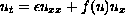,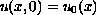,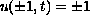(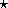)
on the bounded spatial domain [-1,1];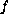is a smooth function satisfying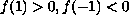and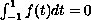. The initial and boundary value problem () has a unique asymptotically stable equilibrium solution that attracts all solutions starting with continuous initial data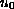. On the infinite spatial domain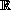the differential equation has slow speed traveling wave solutions generated by profiles that satisfy the boundary conditions of (). As long as its zero stays inside the interval [-1,1], such a traveling wave suitably describes the slow long term behaviour of the solution of () and its speed characterizes the local velocity of the slow motion with exponential precision. A solution that starts near a traveling wave moves in a small neighborhood of the traveling wave with exponentially slow velocity (measured as the speed of the unique zero) during an exponentially long time interval (0,T). In this paper we give a unified treatment of the problem, using both Hilbert space and maximum principle methods, and we give rigorous proofs of convergence of the solution and of the asymptotic estimate of the velocity.

Submitted March 10, 1998. Published November 20, 1998.
Math Subject Classification: 35B25 35K60.
Key Words: Slow motion, singular perturbations, exponential precision, Burgers' equation.

Show me the PDF file (326K), TEX file, and other files for this article.

P. P. N. de Groen
Vrije Universiteit Brussel, Department of Mathematics
Pleinlaan 2,
B--1050, Brussels, Belgium
E-mail: pdegroen@vub.ac.be